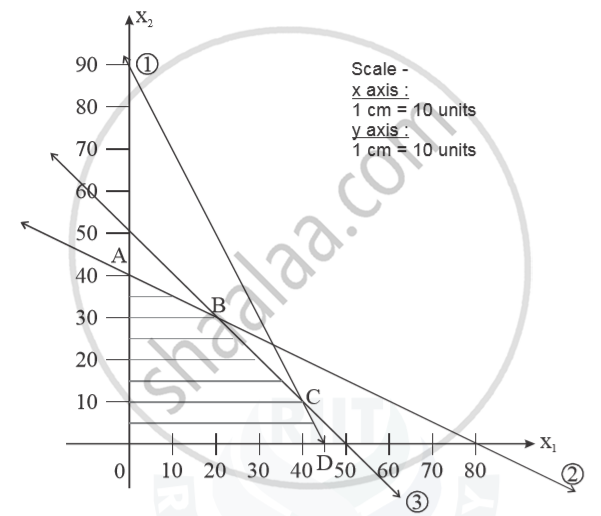Advertisement Remove all ads

# A Carpenter Has 90, 80 and 50 Running Feet Respectively of Teak Wood, Plywood and Rosewood Which is Used to Product a and Product - Mathematics

Sum

A carpenter has 90, 80 and 50 running feet respectively of teak wood, plywood and rosewood which is used to product A and product B. Each unit of product A requires 2, 1 and 1 running feet and each unit of product B requires 1, 2 and 1 running feet of teak wood, plywood and rosewood respectively. If product A is sold for Rs. 48 per unit and product B is sold for Rs. 40 per unit, how many units of product A and product B should be produced and sold by the carpenter, in order to obtain the maximum gross income? Formulate the above as a Linear Programming Problem and solve it, indicating clearly the feasible region in the graph.

Advertisement Remove all ads

#### Solution

 Product A B Teak wood 2 1 ≤90 plywood 1 2 ≤80 Rosewood 1 1 ≤50

Selling price per unit Rs. 48/- for product A and for product B Rs. 40/Mathematical formation :
Z_max = 48x_1 + 40x_2        (objective function)

s.t.      2x1 + x2 ≤ 90
x1 + 2x2 ≤ 80:
x1 + x2 ≤ 50;
x1,x2 ≥ 0
2x1 + x2 = 90           ...........(1)
x1 + 2x2 = 80          ..........(2)
X1 + x2 =50             ........(3)
from eq (3) and (2)
x2 = 30

x1 = 20
from eq. (1) & (3)
x1 = 40
x2 = 10

0(0,0)

 A(0,40) Zmax = 48 × x1 + 40 × x2 ZA = 1600 B(20,30) = 48 × 20 + 40 × 30 ZB = 2160 C(40,10) = 48 × 40 + 40 +10 Zc = 2320 D(45,0) = 48 × 45 + 40(0) ZD = 2160

40, 10), ZC = 2320.
Hence the optimal solution is x1 = 40,  x2 = 10 (Product A  = 40 units, Product B = 10 units)Is there an error in this question or solution?
Advertisement Remove all ads

#### APPEARS IN

Advertisement Remove all ads
Advertisement Remove all ads
Share
Notifications

View all notifications

Forgot password?